×#### Thank you for registering.

One of our academic counsellors will contact you within 1 working day.

Click to Chat

1800-1023-196

+91-120-4616500

CART 0

• 0

MY CART (5)

Use Coupon: CART20 and get 20% off on all online Study Material

ITEM
DETAILS
MRP
DISCOUNT
FINAL PRICE
Total Price: Rs.

There are no items in this cart.
Continue Shopping• Complete JEE Main/Advanced Course and Test Series
• OFFERED PRICE: Rs. 15,900
• View Details

```Chapter 7: Algebraic Expressions Exercise – 7.2

Question: 1

(i) 3x and 7x

(ii) -5xy and 9xy

Solution:

We have

(i) 3x + 7x = (3 + 7) x = 10x

(ii) -5xy + 9xy = (-5 + 9)xy = 4xy

Question: 2

Simplify each of the following:

(i) 7x3y +9yx3

(ii) 12a2b + 3ba2

Solution:

Simplifying the given expressions, we have

(i) 7x3y + 9yx3 = (7 + 9)x3y = 16x3y

(ii) 12a2b + 3ba2 = (12 + 3)a2b =15a2b

Question: 3

(i) 7abc, -5abc, 9abc, -8abc

(ii) 2x2y, - 4x2y, 6x2y, -5x2y

Solution:

Adding the given terms, we have

(i) 7abc + (-5abc) + (9abc) + (-8abc)

= 7abc – 5abc + 9abc – 8abc

= (7 – 5 + 9 – 8)abc

= (16 – 13)abc

= 3abc

(ii) 2x2y +(-4x2y) + (6x2y) + (-5x2y)

= 2x2y - 4x2y + 6x2y - 5x2y

= (2- 4 + 6 - 5) x 2y

= (8 - 9) x 2y

= -x2y

Question: 4

(i) x3 -2x2y + 3xy2- y3, 2x3- 5xy2 + 3x2y - 4y3

(ii) a4 - 2a3b + 3ab3 + 4a2b2 + 3b4, - 2a4 - 5ab3 + 7a3b - 6a2b2 + b4

Solution:

Adding the given expressions, we have

(i) x3 -2x2y + 3xy2- y3, 2x3- 5xy2 + 3x2y - 4y3

Collecting positive and negative like terms together, we get

x3 +2x3 - 2x2y + 3x2y + 3xy2 - 5xy2 - y3- 4y3

= 3x3 + x2y - 2xy2 - 5y3

(ii) a4 - 2a3b + 3ab3 + 4a2b2 + 3b4, - 2a4 - 5ab3 + 7a3b - 6a2b2 + b4

a4 - 2a3b + 3ab3 + 4a2b2 + 3b4 - 2a4 - 5ab3 + 7a3b - 6a2b2 + b4

Collecting positive and negative like terms together, we get

a4 - 2a4- 2a3b + 7a3b + 3ab3 - 5ab3 + 4a2b2 - 6a2b2 + 3b4 + b4

= - a4 + 5a3b - 2ab3 - 2a2b2 + 4b4

Question: 5

(i) 8a – 6ab + 5b, –6a – ab – 8b and –4a + 2ab + 3b

(ii) 5x3 + 7 + 6x - 5x2, 2x2 – 8 - 9x, 4x - 2x2 + 3 x 3, 3 x 3 - 9x - x2 and x - x2 - x3 - 4

Solution:

(i) Required expression = (8a – 6ab + 5b) + (–6a – ab – 8b) + (–4a + 2ab + 3b)

Collecting positive and negative like terms together, we get

8a – 6a – 4a – 6ab – ab + 2ab + 5b – 8b + 3b

= 8a – 10a – 7ab + 2ab + 8b – 8b

= –2a – 5ab

(ii) Required expression = (5 x 3 + 7+ 6x - 5x2) + (2 x 2 – 8 - 9x) + (4x - 2x2 + 3 x 3) + (3 x 3 - 9x-x2) + (x - x2 - x3 - 4)

Collecting positive and negative like terms together, we get

5x3 + 3x3 + 3x3 - x3 - 5x2 + 2x2 - 2x2- x2 - x2 + 6x - 9x + 4x - 9x + x + 7 – 8 - 4

= 10x3 - 7x2 - 7x - 5

Question: 6

(i) x – 3y – 2z

5x + 7y – 8z

3x – 2y + 5z

(ii) 4ab – 5bc + 7ca

–3ab + 2bc – 3ca

5ab – 3bc + 4ca

Solution:

(i) Required expression = (x – 3y – 2z) + (5x + 7y – 8z) + (3x – 2y + 5z)

Collecting positive and negative like terms together, we get

x + 5x + 3x – 3y + 7y – 2y – 2z – 8z + 5z

= 9x – 5y + 7y – 10z + 5z

= 9x + 2y – 5z

(ii) Required expression = (4ab – 5bc + 7ca) + (–3ab + 2bc – 3ca) + (5ab – 3bc + 4ca)

Collecting positive and negative like terms together, we get

4ab – 3ab + 5ab – 5bc + 2bc – 3bc + 7ca – 3ca + 4ca

= 9ab – 3ab – 8bc + 2bc + 11ca – 3ca

= 6ab – 6bc + 8ca

Question: 7

Add 2x2 - 3x + 1 to the sum of 3x2 - 2x and 3x + 7.

Solution:

Sum of 3x2 - 2x and 3x + 7

= (3x2 - 2x) + (3x +7)

=3x2 - 2x + 3x + 7

= (3x2 + x + 7)

Now, required expression = 2x2 - 3x + 1+ (3x2 + x + 7)

= 2x2 + 3x2 - 3x + x + 1 + 7

= 5x2 - 2x + 8

Question: 8

Add x2 + 2xy + y2 to the sum of x2 - 3y2and 2x2 - y2 + 9.

Solution:Question: 9

Add a3+ b3 - 3 to the sum of 2a3 - 3b3 - 3ab + 7 and -a3 + b3 + 3ab - 9.

Solution:Question: 10

Subtract:

(i) 7a2b from 3a2b

(ii) 4xy from -3xy

Solution:

(i) Required expression = 3a2b -7a2b

= (3 -7)a2b

= - 4a2b

(ii) Required expression = –3xy – 4xy

= –7xy

Question: 11

Subtract:

(i) - 4x from 3y

(ii) - 2x from – 5y

Solution:

(i) Required expression = (3y) – (–4x)

= 3y + 4x

(ii) Required expression = (-5y) – (–2x)

= –5y + 2x

Question: 12

Subtract:

(i) 6x3 −7x2 + 5x − 3 from 4 − 5x + 6x2 − 8x3

(ii) − x2 −3z from 5x2 – y + z + 7

(iii) x3 + 2x2y + 6xy2 − y3 from y3−3xy2−4x2y

Solution:Question: 13

From

(i) p3 – 4 + 3p2, take away 5p2 − 3p3 + p − 6

(ii) 7 + x − x2, take away 9 + x + 3x2 + 7x3

(iii) 1− 5y2, take away y3 + 7y2 + y + 1

(iv) x3 − 5x2 + 3x + 1, take away 6x2 − 4x3 + 5 + 3x

Solution: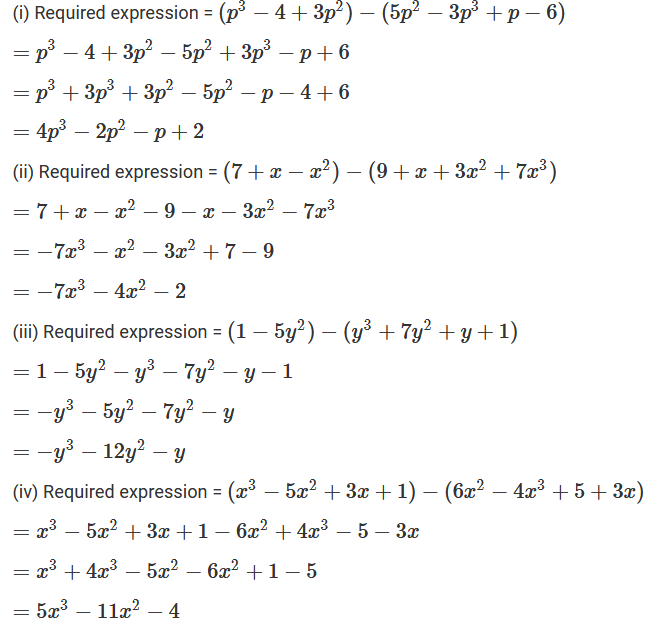Question: 14

From the sum of 3x2 − 5x + 2 and − 5x2 − 8x + 9 subtract 4x2 − 7x + 9.

Solution:Question: 15

Subtract the sum of 13x – 4y + 7z and – 6z + 6x + 3y from the sum of 6x – 4y – 4z and   2x + 4y – 7.

Solution:

Sum of (13x – 4y + 7z) and (–6z + 6x + 3y)

= (13x – 4y + 7z) + (–6z + 6x + 3y)

= (13x – 4y + 7z – 6z + 6x + 3y)

= (13x + 6x – 4y + 3y + 7z – 6z)

= (19x – y + z)

Sum of (6x – 4y – 4z) and (2x + 4y – 7)

= (6x – 4y – 4z) + (2x + 4y – 7)

= (6x – 4y – 4z + 2x + 4y – 7)

= (6x + 2x – 4z – 7)

= (8x – 4z – 7)

Now, required expression = (8x – 4z – 7) – (19x – y + z)

= 8x – 4z – 7 – 19x + y – z

= 8x – 19x + y – 4z – z – 7

= –11x + y – 5z – 7

Question: 16

From the sum of x2 + 3y2 − 6xy, 2x2 − y2 + 8xy, y2 + 8 and x2 − 3xy subtract −3x2 + 4y2 – xy + x – y + 3.

Solution:Question: 17

What should be added to xy – 3yz + 4zx to get 4xy – 3zx + 4yz + 7?

Solution:

The required expression can be got by subtracting xy – 3yz + 4zx from 4xy – 3zx + 4yz + 7.

Therefore, required expression = (4xy – 3zx + 4yz + 7) – (xy – 3yz + 4zx)

= 4xy – 3zx + 4yz + 7 – xy + 3yz – 4zx

= 4xy – xy – 3zx – 4zx + 4yz + 3yz + 7

= 3xy – 7zx + 7yz + 7

Question: 18

What should be subtracted from x2 – xy + y2 – x + y + 3 to obtain −x2 + 3y2 − 4xy + 1?

Solution:Question: 19

How much is x – 2y + 3z greater than 3x + 5y – 7?

Solution:

Required expression = (x – 2y + 3z) – (3x + 5y – 7)

= x – 2y + 3z – 3x – 5y + 7

Collecting positive and negative like terms together, we get

x – 3x – 2y + 5y + 3z + 7

= –2x – 7y + 3z + 7

Question: 20

How much is x2 − 2xy + 3y2 less than 2x2 − 3y2 + xy?

Solution: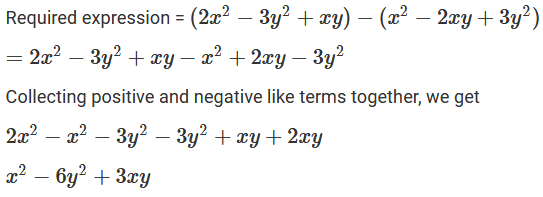Question: 21

How much does a2 − 3ab + 2b2 exceed 2a2 − 7ab + 9b2?

Solution: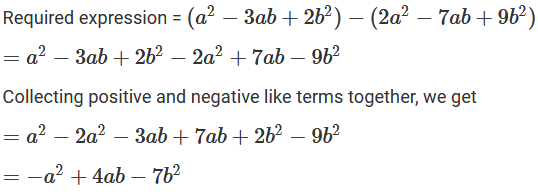Question: 22

What must be added to 12x3 − 4x2 + 3x − 7 to make the sum x3 + 2x2 − 3x + 2?

Solution: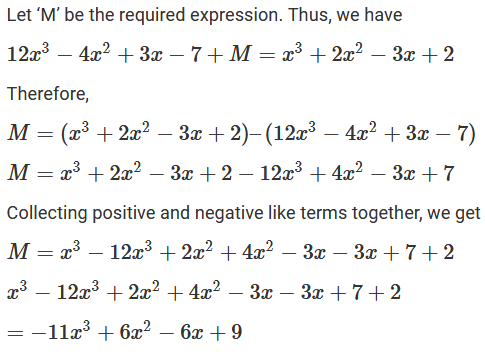Question: 23

If P = 7x2 + 5xy − 9y2, Q = 4y2 − 3x2 − 6xy and R = −4x2 + xy + 5y2, show that P + Q + R = 0.

Solution: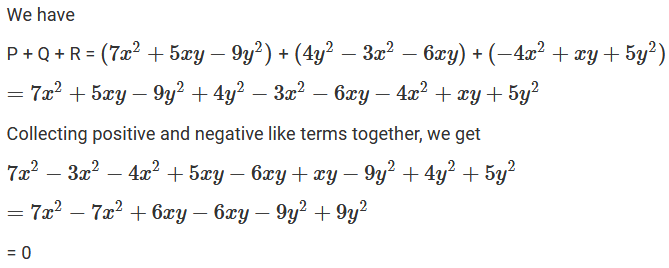Question: 24

If P = a2 − b2 + 2ab, Q = a2 + 4b2 − 6ab, R = b2 + b, S = a2 − 4ab and T = −2a2 + b2 – ab + a. Find P + Q + R + S – T.

Solution: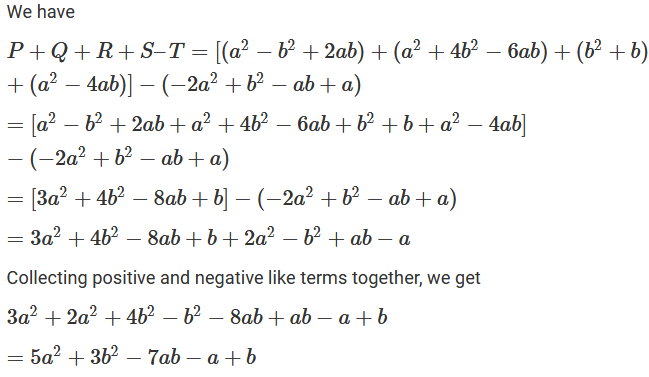```### Course Features

• 728 Video Lectures
• Revision Notes
• Previous Year Papers
• Mind Map
• Study Planner
• NCERT Solutions
• Discussion Forum
• Test paper with Video Solution